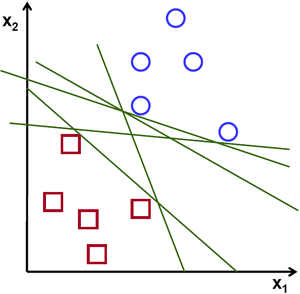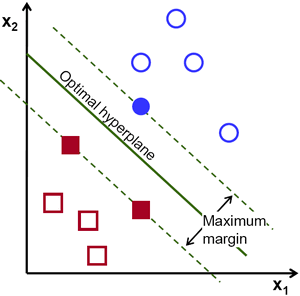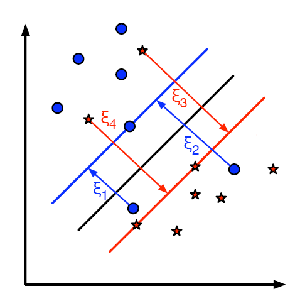# OpenCV-Python 理解SVM | 五十五

### 目标

• 我们将对SVM有一个直观的了解

### 理论

#### 线性可分数据$\min_{w, b_0} L(w, b_0) = \frac{1}{2}||w||^2 \; \text{subject to} \; t_i(w^Tx+b_0) \geq 1 \; \forall i$

### 非线性可分数据

$ϕ(p)=(p^2_1,p^2_2,\sqrt{2}p_1p_2)ϕ(q)=(q^2_1,q^2_2,\sqrt{2}q_1q_2)$让我们定义一个核函数$K(p,q)$，该函数在两点之间做一个点积，如下所示：

\begin{aligned} K(p,q) = \phi(p).\phi(q) &= \phi(p)^T \phi(q) \\ &= (p_{1}^2,p_{2}^2,\sqrt{2} p_1 p_2).(q_{1}^2,q_{2}^2,\sqrt{2} q_1 q_2) \\ &= p_1 q_1 + p_2 q_2 + 2 p_1 q_1 p_2 q_2 \\ &= (p_1 q_1 + p_2 q_2)^2 \\ \phi(p).\phi(q) &= (p.q)^2 \end{aligned}$\min_{w, b_{0}} L(w,b_0) = ||w||^{2} + C \sum_{i} {\xi_{i}} \text{ subject to } y_{i}(w^{T} x_{i} + b_{0}) \geq 1 - \xi_{i} \text{ and } \xi_{i} \geq 0 \text{ } \forall i$

• C的值越大，解决方案的分类错误越少，但宽度也越小。考虑到在这种情况下，进行错误分类错误是昂贵的。由于优化的目的是最小化参数，因此几乎没有误分类的错误。
• C的值越小，解决方案的宽度就越大，分类误差也越大。在这种情况下，最小化对总和项的考虑不多，因此它更多地集中在寻找具有大间隔的超平面上。

## 附加资源

1. NPTEL notes on Statistical Pattern Recognition, Chapters 25-29.

### 练习

http://panchuang.net/

OpenCV中文官方文档：
http://woshicver.com/

http://docs.panchuang.net/

©️2019 CSDN 皮肤主题: 大白 设计师: CSDN官方博客